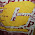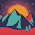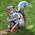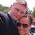Friday, March 25, 2016

Week 10

Blogsheet week 10

In this week’s lab, you will collect more data on low pass and high pass filters and “process” them using MATLAB.
PART A: MATLAB practice.
1.       Open MATLAB. Open the editor and copy paste the following code. Name your code as FirstCode.m
Save the resulting plot as a JPEG image and put it here.
clear all;
close all;
x = [1 2 3 4 5];
y = 2.^x;
plot(x, y, 'LineWidth', 6)
xlabel('Numbers', 'FontSize', 12)
ylabel('Results', 'FontSize', 12)

2.       What does clear all do?
This clears all previous data held in Matlab currently.
3.       What does close all do?
Closes any windows currently open.
4.       In the command line, type x and press enter. This is a matrix. How many rows and columns are there in the matrix?
There are five rows and one column.
5.       Why is there a semicolon at the end of the line of x and y?
This signifies the end of the line of code.
6.       Remove the dot on the y = 2.^x; line and execute the code again. What does the error message mean?
“Error using  ^
Inputs must be a scalar and a square matrix.
To compute elementwise POWER, use POWER (.^) instead.”

7.       How does the LineWidth affect the plot? Explain.
It makes the width of the plot line wider.  For example, the original line width was 6.  The following image is the same plot using a line width of 30.

8.       Type help plot on the command line and study the options for plot command. Provide how you would change the line for plot command to obtain the following figure (Hint: Like ‘LineWidth’, there is another property called ‘MarkerSize’)
We would add “'-or','MarkerSize', 12” to the code.  Where “-or” signifies making the plot red and the ‘MarkerSize, 12” code make the markers large.

9.       What happens if you change the line for x to x = [1; 2; 3; 4; 5]; ? Explain.
Nothing changes.  The graph stays the same both times.
10.   Provide the code for the following figure. You need to figure out the function for y. Notice there are grids on the plot.
11.   Degree vs. radian in MATLAB:
a.       Calculate sinus of 30 degrees using a calculator or internet.
Sin(30) = 0.5
b.      Type sin(30) in the command line of the MATLAB. Why is this number different? (Hint: MATLAB treats angles as radians).
The answer of radians is (-0.9880).  This is because it’s in radians.
c.       How can you modify sin(30) so we get the correct number?
We can convert 30 degrees into radians by multiplying by pi an divide by 180.
12.   Plot y = 10 sin (100 t) using Matlab with two different resolutions on the same plot: 10 points per period and 1000 points per period. The plot needs to show only two periods. Commands you might need to use are linspace, plot, hold on, legend, xlabel, and ylabel. Provide your code and resulting figure. The output figure should look like the following:

clear all;
close all;
t = linspace(0,((4*pi)/100),10);
x = 10*sin(100*t);
f = linspace(0,((4*pi)/100),1000);
y = 10*sin(100*f);

plot(t,x, '-or');
hold on;
plot(f,y);
xlabel('Time (s)', 'FontSize', 12)
ylabel('y function', 'FontSize', 12)

13.   Explain what is changed in the following plot comparing to the previous one.
The fine graph is clipped at a maximum positive amplitude of 5.

14.   The command find was used to create this code. Study the use of find (help find) and try to replicate the plot above. Provide your code.
clear all;
close all;
t = linspace(0,((4*pi)/100),10);
x = 10*sin(100*t);

f = linspace(0,((4*pi)/100),1000);
y = 10*sin(100*f);
y(find(y > 5)) = 5;

plot(t,x, '-or');
hold on;
plot(f,y);
xlabel('Time (s)', 'FontSize', 12)
ylabel('y function', 'FontSize', 12)

15.   Create a code that would clip the negative part of the sinusoidal signal for the fine plot to -5.
clear all;
close all;
t = linspace(0,((4*pi)/100),10);
x = 10*sin(100*t);

f = linspace(0,((4*pi)/100),1000);
y = 10*sin(100*f);
y(find(y < -5)) = -5;

plot(t,x, '-or');
hold on;
plot(f,y);
xlabel('Time (s)', 'FontSize', 12)

ylabel('y function', 'FontSize', 12)

PART B: Filters and MATLAB
1.       Build a low pass filter using a resistor and capacitor in which the cut off frequency is 1 kHz. Observe the output signal using the oscilloscope. Collect several data points particularly around the cut off frequency. Provide your data in a table.

2.       Plot your data using MATLAB. Make sure to use proper labels for the plot and make your plot line and fonts readable. Provide your code and the plot.
x = [5 10 20 30 40 50 100 200 300 400 500 600 700 800 900 1000 1100 1200 1300 1400 2000 3000 4000];
y = [4.72 4.23 3.14 2.4 1.9 1.56 .83 .420 .292 .222 .180 .156 .136 .126 .114 .103 .098 .091 .082 .077 .061 .048 .040];
plot(x, y,'g', 'LineWidth', 6)
xlabel('Frequency ', 'Fontsize', 12)
ylabel('V Peak ','FontSize', 12)
hold on;

3.       Calculate the cut off frequency using MATLAB. find command will be used. Provide your code.

x = [5 10 20 30 40 50 100 200 300 400 500 600 700 800 900 1000 1100 1200 1300 1400 2000 3000 4000];
y = [4.72 4.23 3.14 2.4 1.9 1.56 .83 .420 .292 .222 .180 .156 .136 .126 .114 .103 .098 .091 .082 .077 .061 .048 .040];
plot(x, y,'g', 'LineWidth', 6)
xlabel('Frequency ', 'Fontsize', 12)
ylabel('V Peak ','FontSize', 12)
hold on;
t=find(y==400);
x(t)

4.       Put a horizontal dashed line on the previous plot that passes through the cutoff frequency.

5.       Repeat 1-3 by modifying the circuit to a high pass filter.

x = [100 300 600 900 1200 1500 1800 2100 2400 2700 3000 3300 3600 3900 4200 4500 4800 9000 12000 17000 20000];
y = [0.47 .872 1.47 2.12 3.1 3.89 4.32 4.57 4.82 5.877 6.39 8.11 8.72 8.72 8.72 8.72 8.72 8.72 8.72 8.72 8.72];
plot(x, y, 'LineWidth', 6)
xlabel('Frequency ', 'Fontsize', 12)
ylabel('V Peak ','FontSize', 12)
hold on;

6.       Repeat #4 for #5.

1.I like how on number 7 you have a picture and you made it obvious that there was a change. But you seem to be missing the second half of your blog.

-Ben S.

1.Thanks. I like number 7 too. Just needed to hit the update button.

2.Everything that is there looks solid. Though you are missing half of the blog :/. What was the hardest part about this lab for you?

1.The hardest part seems to have been writing the blog!

3.I would fix up the formatting, it is hard to distinguish the questions from the answers. Also, the code is hard to read as it is now. Maybe take a screenshot when it is in MATLAB?

4.For part A #4 and #9, the matrix x has 1 row and 5 columns if no semi-colon's are placed within the brackets. When semi-colon's are placed within the brackets (like so... x = [1;2;3;4;5]) the matrix x now has 5 rows and 1 column. Therefore, the semi-colon's within the brackets do not affect this graph, but they do affect the matrix x. You can see this within the command window of MATLAB if you type in x with and without the semi-colon's, leaving out the semi-colon at the very end of the line.

5.Nice work, nice big line on question 7 =)

1.Had to make sure it could be seen!

2.Had to make sure it could be seen!

6.Missing parts, yes.